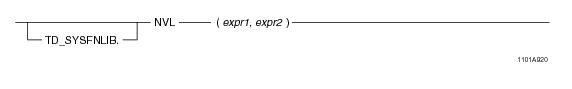# 15.00 - NVL - Teradata Database

## Teradata Database SQL Functions, Operators, Expressions, and Predicates

Product
Release Number
15.00
Content Type
Programming Reference
Publication ID
B035-1145-015K
Language
English (United States)

## NVL

### Purpose

Replaces a NULL with a numeric or a string value as the result value.

### Syntaxwhere:

 Syntax element … Specifies … TD_SYSFNLIB the name of the database where the function is located. expr1 a numeric or character argument. If expr1 is NULL, expr2 is returned. If expr1 is not NULL, expr1 is returned. expr2 a numeric or character argument.

### Invocation

NVL is an embedded services system function. For information on activating and invoking embedded services functions, see “Embedded Services System Functions” on page 24.

### Argument Types and Rules

Expressions passed to this function must have the following data types:

BYTEINT, SMALLINT, INTEGER, BIGINT, DECIMAL/NUMERIC, FLOAT/REAL/DOUBLE PRECISION, NUMBER, CHAR, VARCHAR

All of the input arguments must be the same data type or else the types must be compatible.

### Result Type

NVL is a scalar function whose return value data type depends on the data type associated with the arguments passed to it.

• If the input arguments are numeric types, the function determines which argument has the highest precedence, converts the other argument to that data type, and returns that data type. For details about the order of precedence, see “Compatible Types” in SQL External Routine Programming.
• If that data type is DECIMAL/NUMERIC and the precision and scale of the input arguments are different, the precision and scale of the return type is set to achieve the maximum precision possible. For example, if the input arguments are DECIMAL(6,3), DECIMAL(7,4), and DECIMAL(8,7), the return type would need three digits to the left of the decimal point and seven digits to the right of the decimal point to avoid any reduction in precision.

In cases where it is not possible to maintain the maximum precision, the data is rounded according to the DBS Control Record RoundHalfWayMagUp field. For example, if the input arguments are DECIMAL(32, 8) and DECIMAL(30, 28), the return type will be DECIMAL(38,14). This will allow for 24 digits to the left of the decimal point (required for the DECIMAL(32,8) argument), and 14 digits to the right of the decimal point.

If the data type is fixed point NUMBER and the precision is less than or equal to 38, the precision and scale of the return type are calculated with the same method used for DECIMAL/NUMERIC. However, if the precision is greater than 38, the return type is changed to NUMBER(*) to avoid loss of accuracy. If the data type is floating point NUMBER, the return type is NUMBER(*).

• If the two arguments are character data types, the function converts the second argument to the data type of the first argument and returns the type as VARCHAR.
• If all input character types are LATIN, the result is LATIN. If any input is not LATIN, the function converts all input to Unicode and the return character set is Unicode.
• You can also pass arguments with data types that can be converted to the above types using the implicit data type conversion rules that apply to UDFs.

Note: The UDF implicit type conversion rules are more restrictive than the implicit type conversion rules normally used by Teradata Database. If an argument cannot be converted to the required data type following the UDF implicit conversion rules, it must be explicitly cast.

For details, see “Compatible Types” in SQL External Routine Programming.

### Example

The following query:

`SELECT department_name, `
`   NVL(last_name,'NO EMPLOYEE') "LAST NAME"`
`FROM employee E `
`   FULL OUTER JOIN Department D`
`ON department_number=d.department_number;`
`WHERE department_number IN(402,600);`

returns:

`Department_name      LAST NAME`
`--------- --------------------`
`Software Support     Crane`
`New Department       NO EMPLOYEE`

In this example, the NVL function returned the result as last_name column value from the employee table and for the cases where the last_name value is NULL, the function returned the result as NO EMPLOYEE.